# From the linear model we find expected u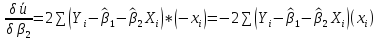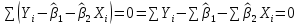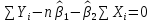; from here we derive β1: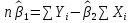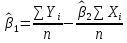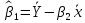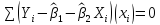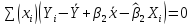; from here we derive β2: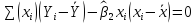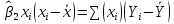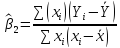According to the variance definition,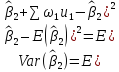as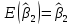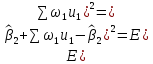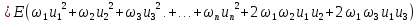Assumptions depicts that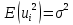; and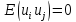; and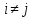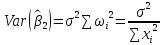The variance of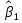can be found using the same way: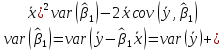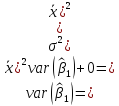The assumptions of Ordinary Least Square (OLS) model: Assumption 1: Linear regression model (in parameters) means that the model’s coefficient must be in the degree of one. Assumption 2: X values are fixed in repeated sampling, which means that regressor must be nonstochastic. Assumption 3: Zero mean value of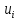: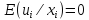, the value of the random disturbance term (error term) is zero. Assumption 4: Homoscedasticity or equal variance of: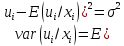Theoretically, the equation characterizes the assumption of homoscedasticity, or equal variance. In other words, it means that the Y populations corresponding to various X values have the same variance. Assumption 5: No autocorrelation between the disturbances: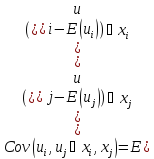WithThe disturbances u­i and uj are uncorrelated, i.e., no serial correlation. This means that, given xi, the deviations of any two Y values from their mean value do not exhibit patterns. Assumption 6: Zero covariance between ui and Xi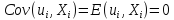The error term u and right-hand side variable X are uncorrelated. Assumption 7: The number of observations n must be greater than the number of parameters to be estimated. Assumption 8: Variability in X values. They must not all be the same. If all values of X is the same, then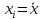, which makes the denominator 0 and calculation of β1 and β2 will be impossible. Assumption 9: The regression model is correctly specified.in other words, the model that is used in empirical analysis must not have specification errors. Assumption 10: There is no perfect multicollinearity between Xs. That is to say, the relationship between independent variables must not be perfect. The followings are the assumptions: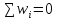;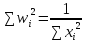;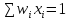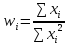;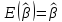;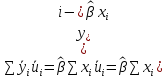; from this equation we found out βhat: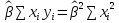;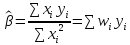;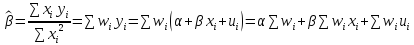;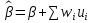;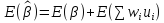;; By using assumption we prove that the coefficient of OLS is unbiasedDownload 0.84 Mb.Do'stlaringiz bilan baham: